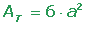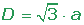Chapters

A cube or regular hexahedron is a regular polyhedron composed of 6 equal squares.### Unfold of a Cube### Characteristics of a Cube

Number of faces: 6.

Number of vertices: 8.

Number of edges: 12.

Number of concurrent edges at a vertex: 3.

### Surface Area of a Cube### Volume of a Cube### Diagonal of a Cube## Example

Calculate the diagonal, the lateral area, suface area and volume of a cube with an edge of 5 cm.Did you like the article?(1 votes, average: 5.00 out of 5)Loading...

Emma

I am passionate about travelling and currently live and work in Paris. I like to spend my time reading, gardening, running, learning languages and exploring new places.

Did you like
this resource?

Bravo!